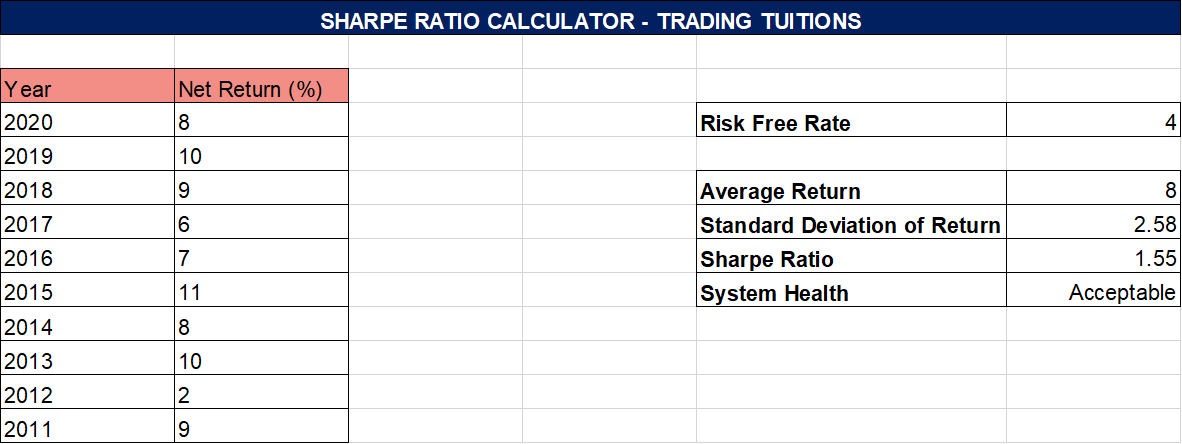# Sharpe Ratio Calculator Excel Sheet

Sharpe Ratio is an indispensable metric to assess the performance of a trading system or a mutual fund. Usually expressed as decimals, it gives an indication of the reliability and sustainability of your investment returns over time. In this post, we’ll understand in-depth about Sharpe ratio and its calculation, also you’ll get a downloadable Sharpe ratio calculator excel sheet to play around with.

## What is Sharpe Ratio?

Sharpe Ratio is the measure of the return of the trading system relative to the risk-free return and volatility. It’s a relative metric which means it would make sense only while comparing two or more systems, individually it doesn’t make much sense.

Developed by Nobel Laureate William F. Sharpe, Sharpe Ratio is one of the most important parameters to judge the performance of your investment. Just relying on absolute returns is not an ideal way to compare trading systems as drawdowns may hit your portfolio from time to time. Sharpe ratio solves this problem by factoring in risk (volatility) along with the return.

The higher the Sharpe ratio, the better is the system. And a higher Sharpe ratio can be achieved by high returns and low volatility.

## Sharpe Ratio Calculation

Below is the formula to calculate Sharpe Ratio:

Sharpe Ratio = (Rp – Rf)/σp

where

Rp = System Return (in %)

Rf = Risk Free return rate (in %).

σp = Standard Deviation (in %)

For risk-free return rate, you may consider US treasury bill yield or Bank fixed deposit rate as a reference. It may vary from 2% to 6% depending on the country you live in.

Both Rp and σp are calculated on a series and not on individual values. For example: if you have recorded your daily returns for the last 30 days, then Rp will be the average of those returns and σp will be the standard deviation of the return %. This would be clear once you see the excel sheet we developed for calculating the Sharpe ratio.

In a nutshell, the Sharpe ratio is calculated by dividing the below two metrics:

1. Excess returns of your system over the risk-free return rate.
2. The standard deviation of the system’s returns over a particular time period.

Let’s take an example:

Suppose the net yearly returns of your trading system over the last 5 years are 15%, 13%, 10%, 12%, and 10%

Rp = (15+13+10+12+10)/5 = 12%

Let’s say the risk-free return is 4%.

Then, Excess return = 12 – 4 = 8%

Now, the standard deviation represents the average variance of each year’s return% from the mean. It is calculated as the square root of variance by determining each data point’s deviation relative to the mean.

In our example, the mean is the average of return %, which is equal to 12

The variance is determined by subtracting the mean’s value from each data point, resulting in 3, 1, -2, 0, and -2. Each of those values is then squared, resulting in 9, 1, 4, 0, and 4. The square values are then added together, giving a total of 18, which is then divided by the value of N minus 1, which is 4, resulting in a variance of approximately 4.5.

The square root of the variance is then calculated, which results in a standard deviation measure of approximately 2.121.

The excess return is divided by standard deviation which results in Sharpe ratio

Sharpe Ratio = 8/2.121 = 3.77

## Sharpe Ratio Calculator Excel Sheet

I hope you now understand that the Sharpe ratio is an important thing to consider while evaluating any trading system. However, its calculation can get complicated, isn’t it?

Calculating it manually would be extremely tedious, hence all modern backtesting engines like Amibroker already have Sharpe ratio embedded in their backtesting report.

However, if you are a spreadsheet person and don’t like to work on other softwares, we have developed a Sharpe ratio calculator excel sheet that you can download and use immediately.

All you need to do is punch in your net return percentages and excel will do the magic for you. For illustration purposes, we have recorded hypothetical yearly return values in the excel, you can change the % as well as time period as per your wish.The risk-free rate is considered as 4% and it can also be changed as required. Standard deviation is calculated using the inbuilt stddev() function in the excel sheet.

The system health tells you how trustworthy is the system:

1 – 1.99 Acceptable

2 – 2.99 Great

More than 3 Excellent